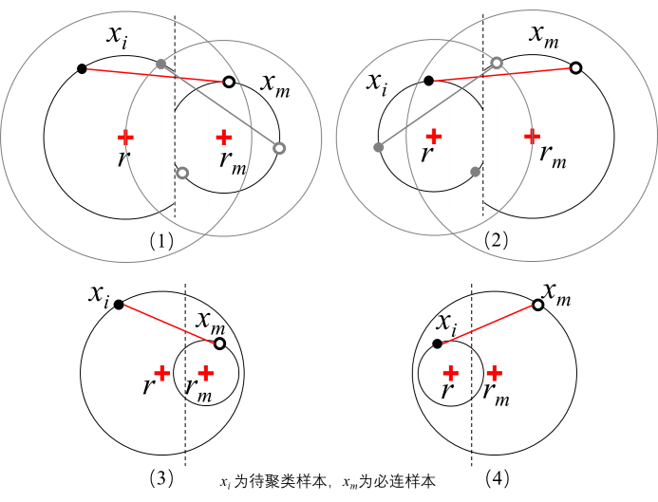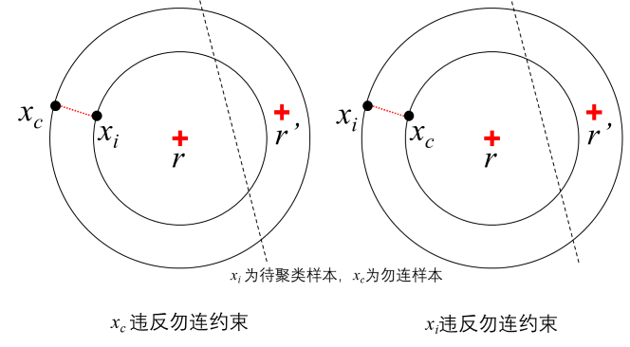# 机器学习-周志华-个人练习13.10

## 13.10 试为图13.7算法第10行写出违约检测算法（用以检测是否有约束未被满足）

### 必连约束违约检测

xirmxmrm>xmrxir,

xirmxmrmxmrxir,

xirmxmrm>xmrxir,

xirmxmrmxmrxir,xirm+xirxmrm+xmr

### 勿连约束违约检测xir>xcr

### 违约检测算法

01: is_violate=False$\mathtt{is\_violate=False}$
02: 找到xi$\mathbf x_i$所属的连通关系集合Xm={xm}$\mathbf X_m=\{\mathbf x_m\}$
03: ifX:$\mathrm {if}\; \mathbf X \ne \varnothing:$
04: forxmXm$\quad \mathrm{for} \; \mathbf x_m \in \mathbf X_m$:
05: Kxmrm=argminjKxmμj$\qquad 基于\mathcal K 找到与\mathbf x_m距离最近的簇r_m=\arg \min_{j \in \mathcal K} \Vert \mathbf x_m-\mu_j\Vert$
06: ifrmrandxmμrm+xmμrxiμrm+xiμr$\qquad \mathrm{if} \; r_m \ne r \; \mathrm{and} \; \Vert \mathbf x_m-\mu_{r_m}\Vert +\Vert \mathbf x_m-\mu_{r}\Vert \ge \Vert \mathbf x_i-\mu_{r_m}\Vert+\Vert \mathbf x_i-\mu_{r}\Vert$:
07: is_violate=True$\qquad \quad \mathtt{is\_violate=True}$
08: break$\qquad \quad \mathrm{break}$
09: if ¬is_violate:$\text{if } \lnot \mathtt{is\_violate}:$
10: xi$\quad 找到\mathbf x_i$所属的勿连关系集合Xc={xc}$\mathbf X_c=\{\mathbf x_c\}$
11: ifX:$\quad \mathrm {if}\; \mathbf X \ne \varnothing:$
12: for xcXc$\qquad \text{for }\mathbf x_c \in \mathbf X_c$:
13: Kxcrc=argminjKxcμj$\qquad \quad 基于\mathcal K 找到与\mathbf x_c距离最近的簇r_c=\arg \min_{j \in \mathcal K} \Vert \mathbf x_c-\mu_j\Vert$
14: if rm=randxcμr<xiμr$\qquad \quad \text{if }r_m = r \; \mathrm{and} \; \Vert \mathbf x_c-\mu_{r}\Vert\lt \Vert \mathbf x_i-\mu_{r}\Vert$:
15: is_violate=True$\qquad \qquad \mathtt{is\_violate=True}$
16: break$\qquad \qquad \mathrm{break}$